# Sketch the V-I characteristics of UJT and describe some of its important applications.

## V-I characteristics of UJT

You can see the V-I characteristics of UJT below. It shows the curve between emitter voltage (VE) and emitter current(IE) of a UJT at a given voltage VBB between the bases.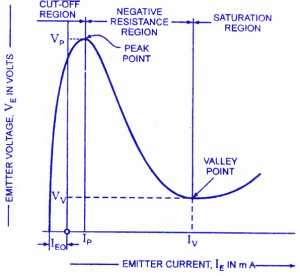Fig.1

The following points may be noted from the characteristics:

(i) Initially, in the cut-off region, as VE  increases from zero, slight leakage current flows from terminal Bto the emitter.

This current is due to the minority carriers in the reverse biased diode.

(ii) Above a certain value of VE, forward Ibegins to flow, increasing until the peak voltage Vand Iare reached at point P.

(iii) After the peak point P, an attempt to increase Vis followed by a sudden increase in emitter current Iwith a corresponding decrease in VE.

This is a negative resistance portion of the curve because with increase in IE, Vdecreases.

The device, therefore, has a negative resistance region which is stable enough to be used with a great deal of reliability in many areas e.g. trigger circuits, saw-tooth generators, timing circuits.

(iv) The negative portion of the curve lasts until the valley point V is reached with valley-point voltage Vand valley-point current IV. After the valley point, the device is driven to saturation.

Fig.2 shows the typical family of VE /IE characteristics of a UJT at different voltages between the bases.

It is clear that peak-point voltage (=ηVBB+VD) falls steadily with reducing VBB and so does the valley-point voltage Vv.

The UJT has achieved great popularity due to the following reasons:

1. It is low cost device.
2. It has excellent characteristics.
3. It is a low-power absorbing device under normal operating conditions.

Due to the above reasons, this device is being used in a variety of applications. A few include oscillators, trigger circuits, saw-tooth generators, bistable network etc.

### Applications of UJT

#### 1.  UJT relaxation oscillator

In a basic and typical UJT relaxation oscillator circuit, the Emitter terminal of the unijunction transistor is connected to the junction of a series connected resistor and capacitor, RC circuit as shown below in Fig.3.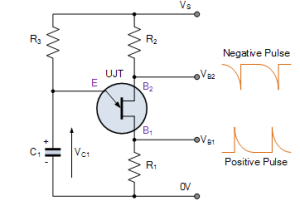Fig.3

When a voltage Vs is turned on, the capacitor C1 charges through resistor R3.

During the charging period, the voltage across the capacitor rises in an exponential manner until it reaches the peak-point voltage.

As the Emitter of the UJT is connected to the capacitor, when the charging voltage Vc across the capacitor C1 becomes greater than the diode volt drop value, the p-n junction behaves as a normal diode and becomes forward biased, triggering the UJT into conduction.

The unijunction transistor is “ON”.

At this point the Emitter to B1 impedance collapses as the Emitter goes into a low impedance saturated state with the flow of Emitter current through R1 taking place.

As the ohmic value of resistor R1 is very low, the capacitor discharges rapidly through the UJT and a fast rising voltage pulse appears across R1.

Also, because the capacitor discharges more quickly through the UJT than it does charging up through resistor R3, the discharging time is a lot less than the charging time as the capacitor discharges through the low resistance UJT.

When the voltage across the capacitor decreases below the holding point of the p-n junction ( VOFF ), the UJT turns “OFF” and no current flows into the Emitter junction so once again the capacitor charges up through resistor R3 and this charging and discharging process between VON and VOFF is constantly repeated while there is a supply voltage, Vs applied.

##### UJT Oscillator WaveformsFig.4

We can see that the unijunction oscillator continually switches “ON” and “OFF” without any feedback.

The output pulse shape generated from the Base1 (B1) terminal is that of a sawtooth waveform and to regulate the time period, you only have to change the ohmic value of resistance, R3 since it sets the RC time constant for charging the capacitor.

The time period, T of the sawtoothed waveform will be given as the charging time plus the discharging time of the capacitor. As the discharge time, τ1 is generally very short in comparison to the larger RC charging time, τ2 the time period of oscillation is more or less equivalent to T ≅ τ2. The frequency of oscillation is therefore given by ƒ = 1/T.

The time period and hence the frequency of the saw-tooth wave can be calculated as follows:

Assuming that the capacitor is initially uncharged, the voltage

The output pulse shape generated from the Base1 (B1) terminal is that of a sawtooth waveform and to regulate the time period, you only have to change the ohmic value of resistance, R3 since it sets the RC time constant for charging the capacitor.

The time period, T of the sawtoothed waveform will be given as the charging time plus the discharging time of the capacitor. As the discharge time, τ1 is generally very short in comparison to the larger RC charging time, τ2 the time period of oscillation is more or less equivalent to T ≅ τ2. The frequency of oscillation is therefore given by ƒ = 1/T.

across the capacitor prior to break-down is given by: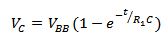Where                    R1C = Charging time constant of resistor-capacitor circuit

t = time from the commencement of waveform

The discharge of the capacitor occurs when Vis equal to the peak-point voltage ηVBB i.e.Or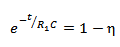Or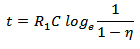Or, time period,

Frequency of saw-tooth wave,

#### 2.  Overvoltage Detector

Fig.5 shows a simple d.c. over-voltage indicator.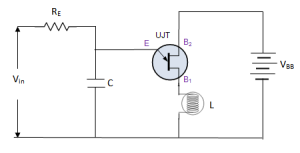Fig.5

A warning pilot-lamp L is connected between the emitter and Bin the circuit.

So long as the input voltage is less than the peak-point voltage(VP) of the UJT, the device remains switched off.

However, when the input voltage exceeds VP, the UJT is switched on and the capacitor discharges through the low resistance path between terminals E and B1.

The current flowing in the pilot lamp L lights it, thereby indicating the over-voltage in the circuit.